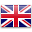Teaching & Learning

# Mathematics

Mathematics is the study of number and space.

It is a search for patterns and relationships that will lead to the development of concepts and generalisations that can be applied in finding solutions to problems, improving our understanding of the world around us and meeting the needs of specific people.  It is a way of thinking characterised by exploring, manipulating, classifying, generalising, abstracting, estimating, calculating, predicting, describing, deducing, drawing and measuring.

Mathematics is a powerful, precise and concise means of communication used to represent, to interpret, to explain and to predict. It is an essential and relevant part of everyday life.

The outcomes of the Mathematics syllabus are divided into strands:• Numbers and Algebra
• Measurement and Geometry
• Statistics and Probability
• Working Mathematically

Teaching and learning of Mathematics at St Patrick’s is based on the NSW Syllabus for the Australian Curriculum.

Students appreciate Mathematics as an essential and relevant part of life and develop their ability to work mathematically.

We encourage positive attitudes towards learning by developing students’ understanding for applying Mathematics effectively to become proficient problem solvers and to value the place of Mathematics in society.

Through our Mathematics program students will:

• develop a sound understanding of mathematical concepts, processes and strategies and the capacity to use these in solving problems
• develop the ability to apply their mathematics to analyse situations and solve real life problems
• develop appropriate language for the effective communication of mathematical ideas and experiences
• develop an appreciation of the applications to mathematics
of technology, including calculators and computers

St Patrick’s Catholic Primary School has mandatory assessment requirements and student tracking in Mathematical learning from Kindergarten to Year Six.  Consistent assessment data analysis from teachers provides valuable information to continually develop student learning from their point of need in Mathematics.English